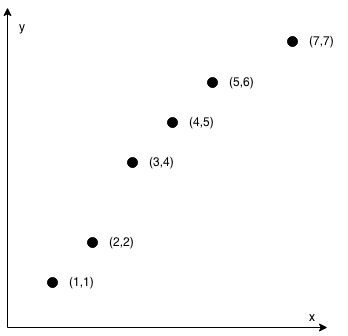# Check If Points Make a Straight Line

Easy
7
70.8% Acceptance

In this lab, you are provided with an array of points `coordinates`, and every element `coordinates[i]` represents a coordinate on a 2D plane. Your goal is to determine whether the given points make a straight line or not. To solve this, you will implement a function `checkStraightLine(coordinates)` that takes an array of coordinates and returns a boolean value `true` if the points form a straight line, and `false` otherwise.

Example 1:

Input: coordinates = [[1,2],[2,3],[3,4],[4,5],[5,6],[6,7]] Output: trueExample 2:

Input: coordinates = [[1,1],[2,2],[3,4],[4,5],[5,6],[7,7]] Output: falseConstraints:

• `2 <= coordinates.length <= 1000`
• `coordinates[i].length == 2`
• `-10^4 <= coordinates[i], coordinates[i] <= 10^4`
• `coordinates` contains no duplicate point.

## Challenges

1. Export the function `checkStraightLine` from `index.js`.
2. Implement the function `checkStraightLine` to return `true` if the points form a straight line, and `false` otherwise.